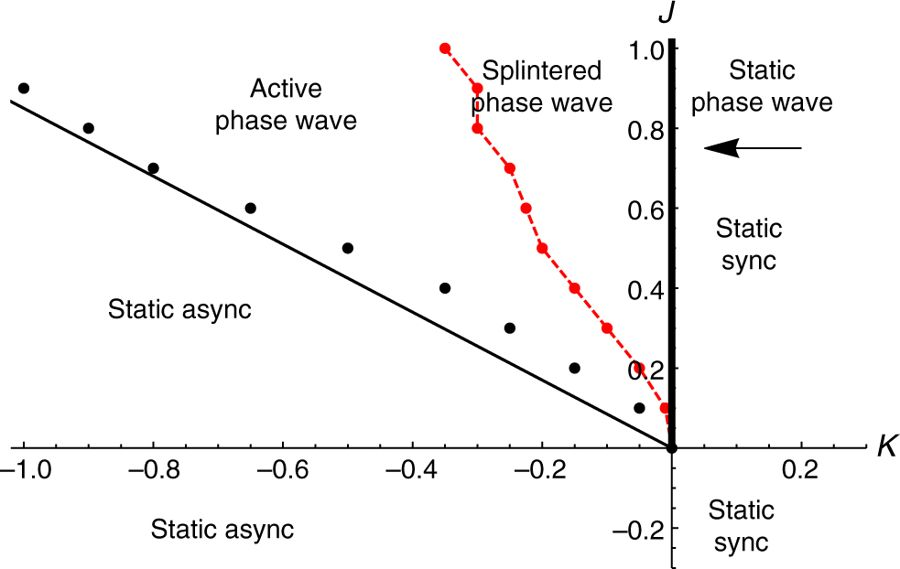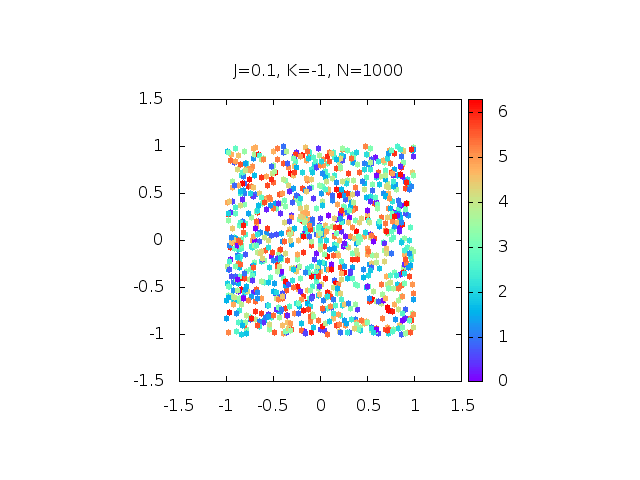# A Storm of Oscillators

Original paper: Oscillators that sync and swarm

Japanese tree frogs follow a mating ritual that is so strange and beautiful that studying them may give rise to a new kind of science. During the rainy season, the male frogs gather near paddies and ponds and call to attract females. Researchers have found that isolated single male frogs call periodically , however, groups of males that are located near each other try to call out of sync. This temporal difference in calls allows the female frogs to correctly locate the male frogs. This is a natural system where spatial location of the frogs dictates the synchronization of their calls. This leads to an interesting question: What happens if you allow the synchronization of their calls to dictate their locations instead?

Scientists study synchronization to understand how an oscillating system evolves over time. However, systems ‘in sync’ do not usually move through space in a way that is related to their oscillations – consider the light flashes from a gathering of fireflies or the beating of a cardiac pacemaker. Swarming, on the other hand, is a form of self-organization where the participating individuals move through space, guided by some simple rules. In today’s paper by O’Keeffe, Hong, and Strogatz, the authors try to get a theoretical understanding of systems that show both synchronization and swarming. Previously, there had been studies of “mobile oscillators” where it was assumed that the location of an oscillator in space affected its phase. Here, the authors come up with a new theory that the phase affects where the oscillator is located in space. They name such particles swarmalators and propose a simple model to study their collective states analytically.

Imagine a set of particles on a plane, where each one is labeled by a number 1,2,3, etc. The ith particle (where i is any of the labels 1,2,3, etc.) has an internal state represented by a variable theta,$\mathbf{\theta}_i$, and a position represented by the vector$\mathbf{x}_i$. The internal state$\mathbf{\theta}_i$ is equivalent to a phase angle, so it can only take on values between 0 and 2?, while$\mathbf{x}_i$ can be any position on the plane. The key to swarmalators is that their position affects how their internal state changes and their internal state affects how their position changes. A relatively simple way to model this would be by the following set of equations:$\dot{\mathbf{x}}_i = \frac{1}{N} \sum_{j \neq i}^{N} \frac{x_j-x_i}{|x_j-x_i|}(1+J cos(\theta_j-\theta_i)) - \frac{x_j-x_i}{|x_j-x_i|^2}$$\dot{\mathbf{\theta_i}} = \frac{K}{N}\sum_{j \neq i}^{N}\frac{sin(\theta_j-\theta_i)}{|x_j-x_i|}$

The most interesting parameters in these equations are J and K. Let’s step through the equations to see what their effects are. The first equation says how the position of the ith swarmalator changes in time, given by the time derivative of$\dot{\mathbf{x}}_i$. It is affected by all the other particles. The first term in the sum is an attraction between particles and points in the direction from particle i to particle j. The parameter J controls how oscillations lead to reorganization in space. For positive J, the closer two swarmalators internal states are, the more they attract since cos(x) is maximized around x = 0. When J is negative, swarmalators are attracted to swarmaltors with as different from an internal state as possible. When J = 0, swaramalators’ spatial movements are immune to other’s internal state. The second term is simply a repulsion between particles, preventing them from occupying the same place.

Similarly, the second equation says how the internal state (the phase angle) changes in time. Like the position, it has a constant angular velocity,$\mathbf{\omega}_i$, and is also affected by all the other particles. The parameter K represents how well the internal state of oscillations are synchronized for swarmalators. For a positive K, the swaramalators want to be synchronized with each other, while for negative K they want to oscillate out of phase. This effect is distance dependent, so swarmalators are more affected by nearby swarmalators than ones that are further away.

The authors use a computer to solve this pair of governing equations to see how such a system will evolve over time. They report that there can be five different states based on the two parameters J and K (Figure 1).Figure 1: Diagram showing the various combinations of J and K which give rise to the various states of the model.

Static synchrony: For all positive K and all J, the model predicts that swarmalators will arrange themselves in a circularly symmetric manner. Each of the swarmalators will be fully synchronized in phase (Figure 2). All static states reach an equilibrium after some time.Figure 2: This animation shows the time evolution of swarmalators when they are in a state of static synchrony. All swarmalators occupy a disk and they all have the same phase.

Static asynchrony: For negative J and negative K, i.e. for cases when swarmalators want to oscillate out of phase but are attracted to opposite phases spatially, they are distributed uniformly and every phase occurs everywhere. (Figure 3).Figure 3: The animation shows how swarmalators evolve and rearrange themselves in the state of static asynchrony. The swarmaltors all occupy a disk, with phases different from their neighbors, leading to all phases existing everywhere.

Static phase wave: For the special case K=0 and J>0,.when the swarmalators’ phases are frozen in time but they like to settle near other swarmalators with the same phase, an interesting phenomenon occurs. Since positive J means “like attracts like” the swarmalators arrange themselves in regions of similar phases. This leads to an annular structure where the spatial angle of each swarmalator is perfectly correlated with its phase of oscillation (Figure 4).Figure 4: The state of static phase wave where the spatial angle of the swarmalators is directly correlated to their phase of oscillation.

Splintered phase wave: In the K<0 half-plane, and when the magnitude of K is not too large, the swarmalators tend to oscillate out of phase weakly while trying to get closer to similar phases and a non-stationary state occurs. Here the particles keep rearranging themselves into disconnected clusters of distinct phases. Unlike the earlier static states, within each cluster, the swarmalators execute small amplitude oscillations in both position and phase about their mean values.